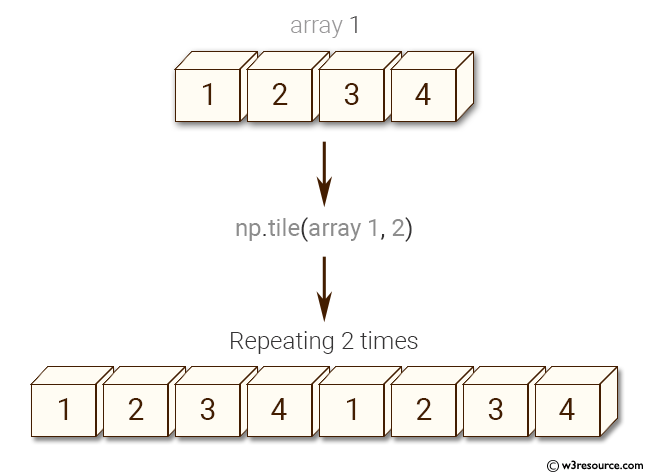﻿ NumPy: Construct an array by repeating - w3resource# NumPy: Construct an array by repeating

## NumPy: Array Object Exercise-25 with Solution

Write a NumPy program to construct an array by repeating.
Sample array: [1, 2, 3, 4]

Pictorial Presentation:Sample Solution:-

Python Code:

``````import numpy as np
a = [1, 2, 3, 4]
print("Original array")
print(a)
print("Repeating 2 times")
x = np.tile(a, 2)
print(x)
print("Repeating 3 times")
x = np.tile(a, 3)
print(x)
```
```

Sample Output:

```Original array
[1, 2, 3, 4]
Repeating 2 times
[1 2 3 4 1 2 3 4]
Repeating 3 times
[1 2 3 4 1 2 3 4 1 2 3 4]
```

Python Code Editor:

Have another way to solve this solution? Contribute your code (and comments) through Disqus.

What is the difficulty level of this exercise?

Test your Python skills with w3resource's quiz

﻿

## Python: Tips of the Day

Set comprehension:

```>>> m = {x ** 2 for x in range(5)}
>>> m
{0, 1, 4, 9, 16}
```